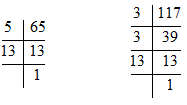#### If the HCF of 65 and 117 is expressible in the form 65 m – 117, then the value of m is (A) 4                (B) 2                (C) 1                (D) 3Solution.   First of all let us find the HCF of 65 and 11765 = 1 × 5 × 13
117 = 1 × 3 × 13 × 3
HCF = 13                    ….(1)
According to question HCF = 65 m – 117
Using (1)
13 = 65 m – 117
13 + 117 = 65 m

m = 2
Therefore, option (b) is correct.